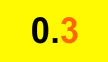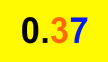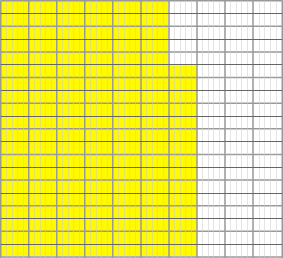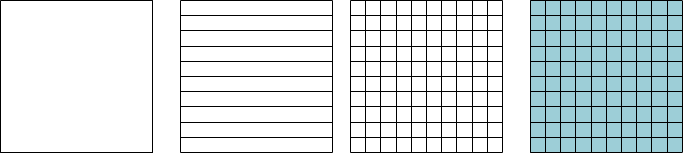# Decimal Illustration

Print Rate 0 stars Common Core
Lesson size:
Message preview:
Someone you know has shared lesson with you:

To play this lesson, click on the link below:

https://www.turtlediary.com/lesson/decimal-illustration.html

Hope you have a good experience with this site and recommend to your friends too.

Login to rate activities and track progress.
Login to rate activities and track progress.

Illustrating decimals with a model helps us better understand just what decimals are. Decimals numbers have place value just as whole numbers do. Remember, with whole numbers that as you move left that whole numbers get ten times greater? So, the place value goes from ones to tens (1 x 10) to hundreds (10 x 10), thousands (100 x 10), etc.Tenths

The value of the first digit to the right of a decimal point is a tenth (0.1). That represents parts of a whole that have been broken into ten equal parts (1/10), like this:

The value of the first digit to the right of a decimal point is a tenth (0.1). That represents parts of a whole that have been broken into ten equal parts

(1/10), like this:

1
2
3
4
5
6
7
8
9
10

Here is the decimal number 0.3 or three tenths.We say "zero point three" when we see 0.3

0.3 means a number has 3 out of 10 parts of a whole.

1
2
3
4
5
6
7
8
9
10
• There is a zero (0) as a placeholder in front of the decimal point to show there is no (or zero) whole numbers in this particular decimal number. In the decimal number 0.3 there are only 3 parts out of 10.
Here are two more examples of decimals with a place value in the tenths:

(a) 0.6

1
2
3
4
5
6
7
8
9
10

six tenths OR 6 out of 10 parts OR We say "zero point six".

(b) 0.9

1
2
3
4
5
6
7
8
9
10

nine tenths OR 9 out of 10 parts OR We say "zero point nine".

Hundredths

Consider the following set: 2, 4, 5, 5, 7

But numbers can be represented as even smaller parts of a whole!

A hundredth (1/10 x 1/10)? ! Hundredths are the next place value to the right. Hundredths represents parts of a whole that have been broken into 100 equal parts.

Hundredths (0.01), therefore, allow us to represent even smaller parts of a whole, like this:

10

20

30

40

50

60

70

80

90

100

Here is the decimal number 0.37 or thirty-seven hundredths.We say "zero point three seven".

0.37 means a number has 37 out of 100 parts of a whole,.

10

20

30
31
32
33
34
35
36
37

40

50

60

70

80

90

100

Here are two more examples of decimals with a place value in the hundredths:

(a) 0.57

10

20

30

40

50
51
52
53
54
55
56
57

60

70

80

90

100

fifty-seven hundredths OR 57 out of 100 parts OR We say "zero point five seven".

10

20

30

40

50
51
52
53
54
55
56
57

60

70

80

90

100

(b) 0.91

10

20

30

40

50

60

70

80

90
91

100

ninety-one hundredths OR 91 out of 100 parts OR We say "zero point nine one"

Thousandths

Decimal numbers allow us to represent as even smaller parts of a whole than a hundredth!

Each hundredth can be broken into ten pieces. So, what is one ten of one hundredth?

A thousandth (1/10 x 1/100=1/1000)?! That makes thousandths the next place value to the right. Thousandths represents parts of a whole that have been broken into 1000 equal parts. Thousandths (.001), therefore, allow us to represent even smaller parts of a whole, like this:Here is the decimal number 0.675 or six hundred seventy-five thousandths.

.We say "zero point six seven five".0.675 means there are 675 out of 1000 parts.

thousandths, millionths, etc. That is a lot of little pieces, much too small to illustrate clearly.

In fact, decimal numbers can keep going further to the right, representing smaller and smaller pieces of a whole, like ten thousandths, hundred Here is a place value model which illustrates the place value of ones, tenths, hundredths and thousandths.## Decimal Illustration

• Decimals numbers have place value just as whole numbers do. Decimals are numbers less than a whole.
• As we move right of the decimal point, the place value gets tens times smaller, from tenths to hundredths to thousandths, and so on.
• Tenths represent parts of a whole broken into ten equal parts (1/10) :
1
2
3
4
5
6
7
8
9
10
• Hundredths are tenths broken into ten equal parts,or 1/10 x 1/10 = 1/100:

10

20

30

40

50

60

70

80

90

100
• Thousandths are hundreds broken into ten equal lparts,or 1/100 x 1/10 = 1/1000:
•• To write a decimal number from a model, look at the total number of parts, and then count the parts of the whole. Place the parts of the whole to the right of a decimal point.

## Similar Lessons

Become premium member to get unlimited access.